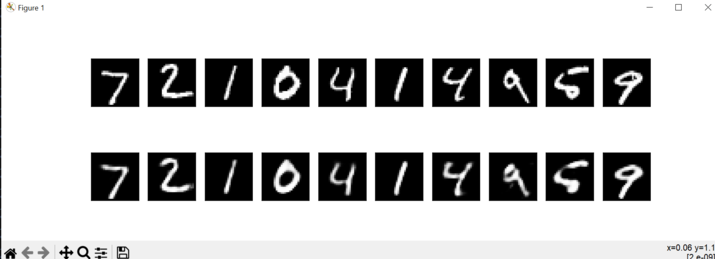# How Autoencoders works?

Autoencoders are a highly effective category of neural networks utilized for unsupervised learning and reducing the dimensionality of data. They possess the ability to learn compact representations of input data by encoding it into a latent space of lower dimensions and subsequently decoding it to restore the original input. This article delves into the workings of autoencoders in Python, specifically employing the Keras library, to provide a comprehensive understanding of their functionality.

## What are autoencoders?

Autoencoders are a specialized form of neural networks designed to reconstruct input data. They consist of two main parts: a decoder network, responsible for recreating the original input from a compressed representation, and an encoder network, which compresses the input into a lower-dimensional representation. Autoencoders excel at recognizing important data features and patterns, and they are trained to minimize errors during the reconstruction process. Their applications include dimensionality reduction, anomaly detection, and model generation.

Data compression, anomaly detection, image synthesis, and denoising are just a few applications where autoencoders are important. They are particularly useful when the input data does not have explicit labels or when we want to extract meaningful features from the data without relying on labeled examples.

## How do autoencoders work?

Autoencoders function by acquiring a compact and effective representation of the input data. To capture essential characteristics, autoencoders employ an encoder network that compresses the input into a latent space of lower dimensions. Subsequently, the decoder network reconstructs the original input from this compressed representation. Throughout the training process, the autoencoder endeavors to minimize the disparity between the input and the reconstructed output. This enables the autoencoder to learn the encoding and decoding of data. By compelling the network to faithfully reconstruct the input, autoencoders gain the ability to derive meaningful representations and extract valuable features. Consequently, they prove valuable in tasks such as reducing dimensionality, detecting anomalies, and generating models.

Below are the steps that we will follow to understand how autoencoders work with a program example −

• Define the autoencoder model with encoder and decoder layers.

• Create separate encoder and decoder models to isolate their functionalities.

• Compile and train the autoencoder on the training data.

• Use the encoder to encode the input test data and the decoder to decode the encoded data back to the original input space after we are done with training the model.

• Visualize the side of the original and the image that is reconstructed using Matplotlib.

The program below will display a figure with 10 rows, where each row contains an original image followed by its reconstructed counterpart. We can also experiment with different hyperparameters and observe how they affect the quality of the reconstructed images.

### Example

import numpy as npp
import matplotlib.pyplot as pltt
from keras.datasets import mnist
from keras.layers import Input, Dense
from keras.models import Model

(x_train_m, _), (x_test_m, _) = mnist.load_data()

# Normalize the pixel values between 0 and 1
x_train_m = x_train_m.astype('float32') / 255.
x_test_m = x_test_m.astype('float32') / 255.

# Reshape the input images
x_train = x_train_m.reshape((len(x_train_m), npp.prod(x_train_m.shape[1:])))
x_test = x_test_m.reshape((len(x_test_m), npp.prod(x_test_m.shape[1:])))

# Define the size of the latent space
latent_dim = 32

# Define the input layer
input_img = Input(shape=(784,))

# Define the encoder layers
encoded1 = Dense(128, activation='relu')(input_img)
encoded2 = Dense(latent_dim, activation='relu')(encoded1)

# Define the decoder layers
decoded1 = Dense(128, activation='relu')(encoded2)
decoded2 = Dense(784, activation='sigmoid')(decoded1)

# Create the autoencoder model
autoencoder = Model(input_img, decoded2)

# Create separate encoder and decoder models
encoder = Model(input_img, encoded2)

# Define the decoder input
latent_input_m = Input(shape=(latent_dim,))
decoder_layer_m = autoencoder.layers[-2](latent_input_m)
decoder_layer_m = autoencoder.layers[-1](decoder_layer_m)
decoder = Model(latent_input_m, decoder_layer_m)

# Compile the autoencoder

# Train the autoencoder
autoencoder.fit(x_train, x_train,
epochs=50,
batch_size=256,
shuffle=True,
validation_data=(x_test, x_test))

# Encode and decode the input test data
encoded_imgs = encoder.predict(x_test)
decoded_imgs = decoder.predict(encoded_imgs)

# Display the original and reconstructed images
n = 10
pltt.figure(figsize=(20, 4))
for i in range(n):
# Original image
ax = pltt.subplot(2, n, i+1)
pltt.imshow(x_test[i].reshape(28, 28))
pltt.gray()
ax.get_xaxis().set_visible(False)
ax.get_yaxis().set_visible(False)

# Reconstructed image
ax = pltt.subplot(2, n, i+n+1)
pltt.imshow(decoded_imgs[i].reshape(28, 28))
pltt.gray()
ax.get_xaxis().set_visible(False)
ax.get_yaxis().set_visible(False)
pltt.show()


### Output## Conclusion

In conclusion, autoencoders provide a powerful framework for learning compressed representations of input data. By employing an encoder-decoder architecture, they can effectively capture important features and patterns within the data. Autoencoders are widely used for tasks such as anomaly detection, dimensionality reduction, and generative modeling. Through the training process, they optimize the reconstruction error, enabling them to learn meaningful representations and extract valuable insights from complex datasets. With their ability to encode and decode data, autoencoders offer a versatile and effective tool for various machine-learning applications.

Updated on: 24-Jul-2023

38 Views